# How to VLOOKUP across multiple sheets in Excel with examples

The tutorial shows how to use the VLOOKUP function to copy data from another worksheet or workbook, Vlookup in multiple sheets, and look up dynamically to return values from different sheets into different cells.

When looking up some information in Excel, it's a rare case when all the data is on the same sheet. More often, you will have to search across multiple sheets or even different workbooks. The good news is that Microsoft Excel provides more than one way to do this, and the bad news is that all the ways are a bit more complicated than a standard VLOOKUP formula. But with just a little patience, we will figure them out :)

## How to VLOOKUP between two sheets

For starters, let's investigate a simplest case - using VLOOKUP to copy data from another worksheet. It's very similar to a regular VLOOKUP formula that searches on the same worksheet. The difference is that you include the sheet name in the table_array argument to tell your formula in which worksheet the lookup range is located.

The generic formula to VLOOKUP from another sheet is as follows:

VLOOKUP(lookup_value, Sheet!range, col_index_num, [range_lookup])

As an example, let's pull the sales figures from Jan report to Summary sheet. For this, we define the following arguments:

• Lookup_values are in column A on the Summary sheet, and we refer to the first data cell, which is A2.
• Table_array is the range A2:B6 on the Jan sheet. To refer to it, prefix the range reference with the sheet name followed by the exclamation point: Jan!\$A\$2:\$B\$6.

Please pay attention that we lock the range with absolute cell references to prevent it from changing when copying the formula to other cells.
Col_index_num is 2 because we want to copy a value from column B, which is the 2nd column in the table array.

• Range_lookup is set to FALSE to look up an exact match.

Putting the arguments together, we get this formula:

`=VLOOKUP(A2, Jan!\$A\$2:\$B\$6, 2, FALSE)`

Drag the formula down the column and you will get this result: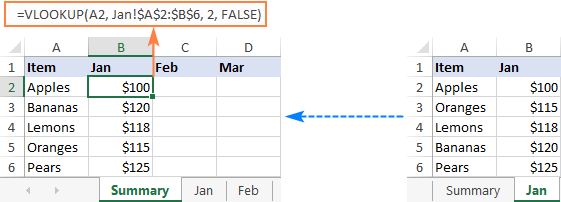In a similar manner, you can Vlookup data from the Feb and Mar sheets:

`=VLOOKUP(A2, Feb!\$A\$2:\$B\$6, 2, FALSE)`

`=VLOOKUP(A2, Mar!\$A\$2:\$B\$6, 2, FALSE)`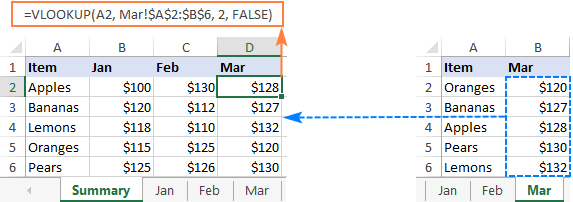Tips and notes:

• If the sheet name contains spaces or non-alphabetical characters, it must be enclosed in single quotation marks, like 'Jan Sales'!\$A\$2:\$B\$6. For more info, please see How to reference another sheet in Excel.
• Instead of typing a sheet name directly in a formula, you can switch to the lookup worksheet and select the range there. Excel will insert a reference with the correct syntax automatically, sparing you the trouble to check the name and troubleshoot.

## Vlookup from a different workbook

To VLOOKUP between two workbooks, include the file name in square brackets, followed by the sheet name and the exclamation point.

For example, to search for A2 value in the range A2:B6 on Jan sheet in the Sales_reports.xlsx workbook, use this formula:

`=VLOOKUP(A2, [Sales_reports.xlsx]Jan!\$A\$2:\$B\$6, 2, FALSE)`

For full details, please see VLOOKUP from another workbook in Excel.

## Vlookup across multiple sheets with IFERROR

When you need to look up between more than two sheets, the easiest solution is to use VLOOKUP in combination with IFERROR. The idea is to nest several IFERROR functions to check multiple worksheets one by one: if the first VLOOKUP does not find a match on the first sheet, search in the next sheet, and so on.

To see how this approach works on real-life data, let's consider the following example. Below is the Summary table that we want to populate with the item names and amounts by looking up the order number in West and East sheets: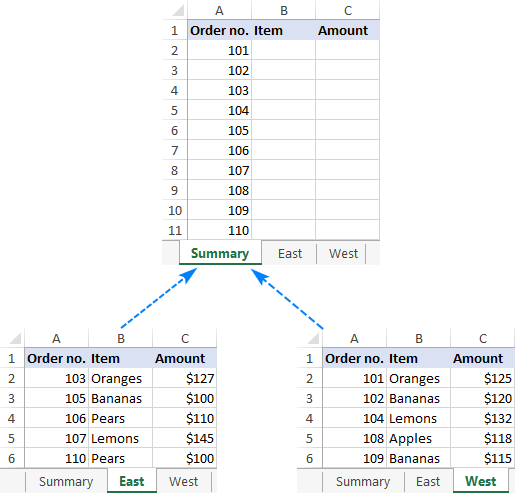First, we are going to pull the items. For this, we instruct the VLOOKUP formula to search for the order number in A2 on the East sheet and return the value from column B (2nd column in table_array A2:C6). If an exact match is not found, then search in the West sheet. If both Vlookups fail, return "Not found".

`=IFERROR(VLOOKUP(A2, East!\$A\$2:\$C\$6, 2, FALSE), IFERROR(VLOOKUP(A2, West!\$A\$2:\$C\$6, 2, FALSE), "Not found"))`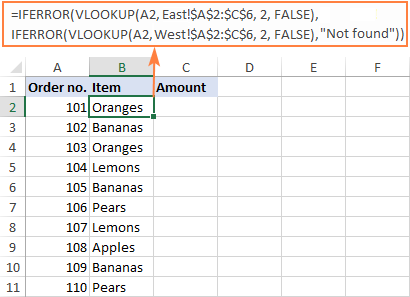To return the amount, simply change the column index number to 3:

`=IFERROR(VLOOKUP(A2, East!\$A\$2:\$C\$6, 3, FALSE), IFERROR(VLOOKUP(A2, West!\$A\$2:\$C\$6, 3, FALSE), "Not found"))`

Tip. If needed, you can specify different table arrays for different VLOOKUP functions. In this example, both lookup sheets have the same number of rows (A2:C6), but your worksheets may be different in size.

### Vlookup in multiple workbooks

To Vlookup between two or more workbooks, enclose the workbook name in square brackets and put it before the sheet name. For example, here's how you can Vlookup in two different files (Book1 and Book2) with a single formula:

`=IFERROR(VLOOKUP(A2, [Book1.xlsx]East!\$A\$2:\$C\$6, 2, FALSE), IFERROR(VLOOKUP(A2, [Book2.xlsx]West!\$A\$2:\$C\$6, 2, FALSE),"Not found"))`

### Make column index number dynamic to Vlookup multiple columns

In situation when you need to return data from several columns, making col_index_num dynamic could save you some time. There are a couple of adjustments to be made:

• For the col_index_num argument, use the COLUMNS function that returns the number of columns in a specified array: COLUMNS(\$A\$1:B\$1). (The row coordinate does not really matter, it can be just any row.)
• In the lookup_value argument, lock the column reference with the \$ sign (\$A2), so it remains fixed when copying the formula to other columns.

As the result, you get a kind of dynamic formula that extracts matching values from different columns, depending on which column the formula is copied to:

`=IFERROR(VLOOKUP(\$A2, East!\$A\$2:\$C\$6, COLUMNS(\$A\$1:B\$1), FALSE), IFERROR(VLOOKUP(\$A2, West!\$A\$2:\$C\$6, COLUMNS(\$A\$1:B\$1), FALSE), "Not found"))`

When entered in column B, COLUMNS(\$A\$1:B\$1) evaluates to 2 telling VLOOKUP to return a value from the 2nd column in the table array.

When copied to column C (i.e. you've dragged the formula from B2 to C2), B\$1 changes to C\$1 because the column reference is relative. Consequently, COLUMNS(\$A\$1:C\$1) evaluates to 3 forcing VLOOKUP to return a value from the 3rd column.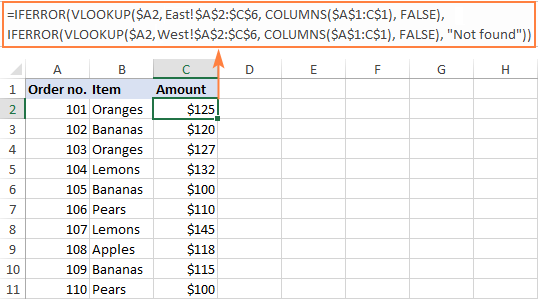This formula works great for 2 - 3 lookup sheets. If you have more, repetitive IFERRORs become too cumbersome. The next example demonstrates a bit more complicated but a lot more elegant approach.

## Vlookup multiple sheets with INDIRECT

One more way to Vlookup between multiple sheets in Excel is to use a combination of VLOOKUP and INDIRECT functions. This method requires a little preparation, but in the end, you will have a more compact formula to Vlookup in any number of spreadsheets.

A generic formula to Vlookup across sheets is as follows:

VLOOKUP(lookup_value, INDIRECT("'"&INDEX(Lookup_sheets, MATCH(1, --(COUNTIF(INDIRECT("'" & Sheet_list & "'!lookup_range"), lookup_value)>0), 0)) & "'!table_array"), col_index_num, FALSE)

Where:

• Lookup_sheets - a named range consisting of the lookup sheet names.
• Lookup_value - the value to search for.
• Lookup_range - the column range in the lookup sheets where to search for the lookup value.
• Table_array - the data range in the lookup sheets.
• Col_index_num - the number of the column in the table array from which to return a value.

For the formula to work correctly, please bear in mind the following caveats:

• It's an array formula, which must be completed by pressing Ctrl + Shift + Enter keys together.
• All the sheets must have the same order of columns.
• As we use one table array for all lookup sheets, specify the largest range if your sheets have different numbers of rows.

### How to use the formula to Vlookup across sheets

To Vlookup multiple sheets at a time, carry out these steps:

1. Write down all the lookup sheet names somewhere in your workbook and name that range (Lookup_sheets in our case).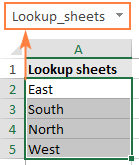2. Adjust the generic formula for your data. In this example, we'll be:
• searching for A2 value (lookup_value)
• in the range A2:A6 (lookup_range) in four worksheets (East, North, South and West), and
• pull matching values from column B, which is column 2 (сol_index_num) in the data range A2:C6 (table_array).

With the above arguments, the formula takes this shape:

`=VLOOKUP(\$A2, INDIRECT("'"&INDEX(Lookup_sheets, MATCH(1, --(COUNTIF(INDIRECT("'"& Lookup_sheets&"'!\$A\$2:\$A\$6"), \$A2)>0), 0)) &"'!\$A\$2:\$C\$6"), 2, FALSE)`

Please notice that we lock both ranges (\$A\$2:\$A\$6 and \$A\$2:\$C\$6) with absolute cell references.

3. Enter the formula in the topmost cell (B2 in this example) and press Ctrl + Shift + Enter to complete it.
4. Double click or drag the fill handle to copy the formula down the column.

As the result, we've got the formula to look up the order number in 4 sheets and retrieve the corresponding item. If a specific order number is not found, a #N/A error is displayed like in row 14: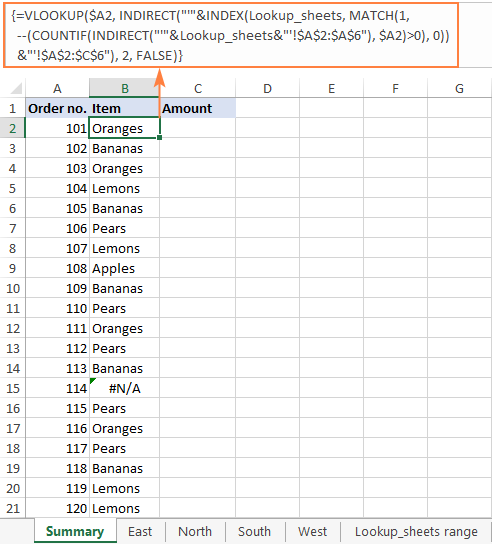To return the amount, simply replace 2 with 3 in the col_index_num argument as amounts are in the 3rd column of the table array:

`=VLOOKUP(\$A2, INDIRECT("'"&INDEX(Lookup_sheets, MATCH(1, --(COUNTIF(INDIRECT("'" & Lookup_sheets & "'!\$A\$2:\$A\$6"), \$A2)>0), 0)) & "'!\$A\$2:\$C\$6"), 3, FALSE)`

If you'd like to replace the standard #N/A error notation with your own text, wrap the formula into the IFNA function:

`=IFNA(VLOOKUP(\$A2, INDIRECT("'"&INDEX(Lookup_sheets, MATCH(1, --(COUNTIF(INDIRECT("'" & Lookup_sheets & "'!\$A\$2:\$A\$6"), \$A2)>0), 0)) & "'!\$A\$2:\$C\$6"), 3, FALSE), "Not found")`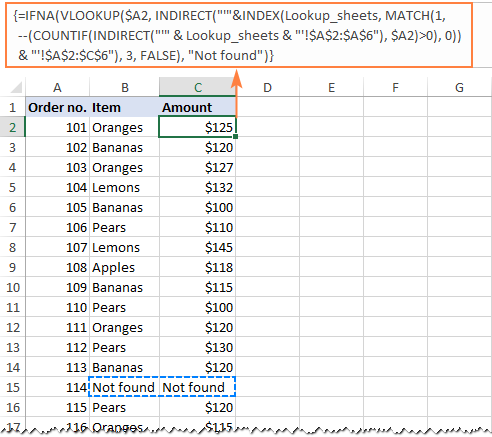### Vlookup multiple sheets between workbooks

This generic formula (or its any variation) can also be used to Vlookup multiple sheets in a different workbook. For this, concatenate the workbook name inside INDIRECT like shown in the below formula:

`=IFNA(VLOOKUP(\$A2, INDIRECT("'[Book1.xlsx]" & INDEX(Lookup_sheets, MATCH(1, --(COUNTIF(INDIRECT("'[Book1.xlsx]" & Lookup_sheets & "'!\$A\$2:\$A\$6"), \$A2)>0), 0)) & "'!\$A\$2:\$C\$6"), 2, FALSE), "Not found")`

### Vlookup between sheets and return multiple columns

If you want to pull data from several columns, a multi-cell array formula can do that in one go. To create such a formula, supply an array constant for the col_index_num argument.

In this example, we wish to return the item names (column B) and amounts (column C), which are the 2nd and 3rd columns in the table array, respectively. So, the required array is {2,3}.

`=VLOOKUP(\$A2, INDIRECT("'"&INDEX(Lookup_sheets, MATCH(1, --(COUNTIF(INDIRECT("'"& Lookup_sheets &"'!\$A\$2:\$C\$6"), \$A2)>0), 0)) &"'!\$A\$2:\$C\$6"), {2,3}, FALSE)`

To correctly enter the formula in multiple cells, this is what you need to do:

• In the first row, select all the cells to be populated (B2:C2 in our example).
• Type the formula and press Ctrl + Shift + Enter. This enters the same formula in the selected cells, which will return a different value in each column.
• Drag down the formula to the remaining rows.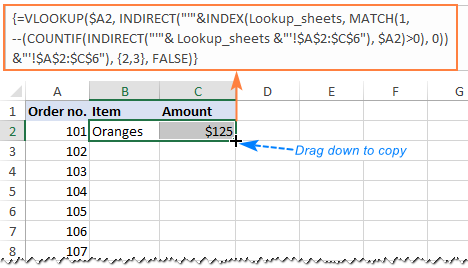### How this formula works

To better understand the logic, let's break down this basic formula to the individual functions:

`=VLOOKUP(\$A2, INDIRECT("'"&INDEX(Lookup_sheets, MATCH(1, --(COUNTIF(INDIRECT("'"& Lookup_sheets&"'!\$A\$2:\$A\$6"), \$A2)>0), 0)) &"'!\$A\$2:\$C\$6"), 2, FALSE)`

Working from the inside out, here's what the formula does:

#### COUNTIF and INDIRECT

In a nutshell, INDIRECT builds the references for all lookup sheets, and COUNTIF counts the occurrences of the lookup value (A2) in each sheet:

`--(COUNTIF( INDIRECT("'"&Lookup_sheets&"'!\$A\$2:\$A\$6"), \$A2)>0)`

In more detail:

First, you concatenate the range name (Lookup_sheets) and the range reference (\$A\$2:\$A\$6), adding apostrophes and the exclamation point in the right places to make an external reference, and feed the resulting text string to the INDIRECT function to dynamically refer to the lookup sheets:

`INDIRECT({"'East'!\$A\$2:\$A\$6"; "'South'!\$A\$2:\$A\$6"; "'North'!\$A\$2:\$A\$6"; "'West'!\$A\$2:\$A\$6"})`

COUNTIF checks each cell in the range A2:A6 on each lookup sheet against the value in A2 on the main sheet and returns the count of matches for each sheet. In our dataset, the order number in A2 (101) is found in the West sheet, which is 4th in the named range, so COUNTIF returns this array:

`{0;0;0;1}`

Next, you compare each element of the above array with 0:

`--({0; 0; 0; 1}>0)`

This yields an array of TRUE (greater than 0) and FALSE (equal to 0) values, which you coerce to 1's and 0's by using a double unary (--), and get the following array as the result:

`{0; 0; 0; 1}`

This operation is an extra precaution to handle a situation when a lookup sheet contains several occurrences of the lookup value, in which case COUNTIF would return a count greater than 1, while we want only 1's and 0's in the final array (in a moment, you will understand why).

After all these transformations, our formula looks as follows:

`VLOOKUP(\$A2, INDIRECT("'"&INDEX(Lookup_sheets, MATCH(1, {0;0;0;1}, 0)) &"'!\$A\$2:\$C\$6"), 2, FALSE)`

#### INDEX and MATCH

At this point, a classic INDEX MATCH combination steps in:

`INDEX(Lookup_sheets, MATCH(1, {0;0;0;1}, 0))`

The MATCH function configured for exact match (0 in the last argument) looks for the value 1 in the array {0;0;0;1} and returns its position, which is 4:

`INDEX(Lookup_sheets, 4)`

The INDEX function uses the number returned by MATCH as the row number argument (row_num), and returns the 4th value in the named range Lookup_sheets, which is West.

So, the formula further reduces to:

`VLOOKUP(\$A2, INDIRECT("'"&"West"&"'!\$A\$2:\$C\$6"), 2, FALSE)`

#### VLOOKUP and INDIRECT

The INDIRECT function processes the text string inside it:

`INDIRECT("'"&"West"&"'!\$A\$2:\$C\$6")`

And converts it into a reference that goes to the table_array argument of VLOOKUP:

`VLOOKUP(\$A2, 'West'!\$A\$2:\$C\$6, 2, FALSE)`

Finally, this very standard VLOOKUP formula searches for the A2 value in the first column of the range A2:C6 on the West sheet and returns a match from the 2nd column. That's it!

## Dynamic VLOOKUP to return data from multiple sheets into different cells

First off, let's define what exactly the word "dynamic" means in this context and how this formula is going to be different from the previous ones.

In case you have large chunks of data in the same format that are split over multiple spreadsheets, you may want to extract information from different sheets into different cells. The image below illustrates the concept: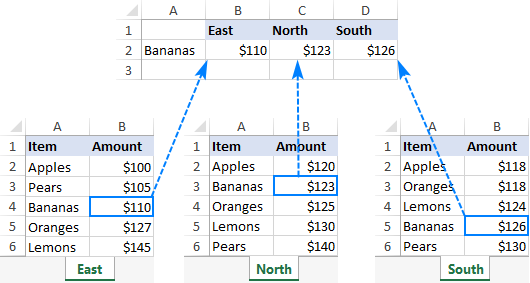Unlike the previous formulas that retrieved a value from a specific sheet based on a unique identifier, this time we are looking to extract values from several sheets at a time.

There are two different solutions for this task. In both cases, you need to do a little preparatory work and create named ranges for data cells in each lookup sheet. For this example, we defined the following ranges:

• East_Sales - A2:B6 on the East sheet
• North_Sales - A2:B6 on the North sheet
• South_Sales - A2:B6 on the South sheet
• West_Sales - A2:B6 on the West sheet

### VLOOKUP and nested IFs

If you have a reasonable number of sheets to look up, you can use nested IF functions to select the sheet based on the keywords in the predefined cells (cells B1 through D1 in our case).

With the lookup value in A2, the formula is follows:

`=VLOOKUP(\$A2, IF(B\$1="east", East_Sales, IF(B\$1="north", North_Sales, IF(B\$1="south", South_Sales, IF(B\$1="west", West_Sales)))), 2, FALSE)`

Translated into English, the IF part reads:

If B1 is East, look in the range named East_Sales; if B1 is North, look in the range named North_Sales; if B1 is South, look in the range named South_Sales; and if B1 is West, look in the range named West_Sales.

The range returned by IF goes to table_array of VLOOKUP, which pulls a matching value from the 2nd column on the corresponding sheet.

The clever use of mixed references for the lookup value (\$A2 - absolute column and relative row) and the logical test of IF (B\$1 - relative column and absolute row) allows copying the formula to other cells without any changes - Excel adjusts the references automatically based on the relative position of a row and column.

So, we enter the formula in B2, copy it right and down to as many columns and rows as needed, and get the following result: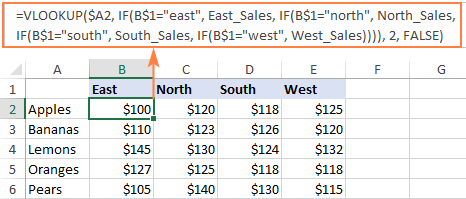### INDIRECT VLOOKUP

When working with many sheets, multiple nested levels could make the formula too lengthy and difficult to read. A far better way is to create a dynamic vlookup range with the help of INDIRECT:

`=VLOOKUP(\$A2, INDIRECT(B\$1&"_Sales"), 2, FALSE)`

Here, we concatenate the reference to the cell that contains a unique part of the named range (B1) and the common part (_Sales). This produces a text string like "East_Sales", which INDIRECT converts to the range name understandable by Excel.

As the result, you get a compact formula that works beautifully on any number of sheets: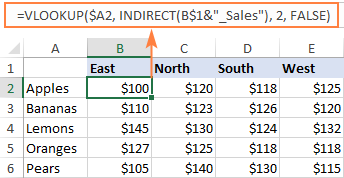That's how to Vlookup between sheets and files in Excel. I thank you for reading and hope to see you on our blog next week!

Vlookup multiple sheets examples (.xlsx file)

### 22 responses to "How to VLOOKUP across multiple sheets in Excel with examples"

1.Sajan Singh says:

Thanks Svetlana & Keep it up Dear.

2.Milosz says:

That is very helpful, bit I am struggling with is how to apply VLOOKUP in only one sheet and return multiple columns if Value I am looking for is in multiple places within row A in that sheet?
Example: I have list of transactions and values one under the other. In row A I got the name of the project that transaction should be allocated and now I have created new sheet and entering formula:
=VLOOKUP(\$A\$1, Sheet1!A:A, {2,3,4,5}, FALSE)
Is there any way for excel to pick up the values in same column and then list them in new sheet made for this Value?

•Svetlana Cheusheva (Ablebits.com Team) says:

Hi Milosz,

No, the VLOOKUP function does not work this way - it can only return one value at a time based on the column index number that you specify. Recently, Microsoft has introduced the XLOOKUP function (currently it's only available to Office 365 subscribers who are part of the Office Insiders program). XLOOKUP is able to return more than one value relating to the same match. Here's an example: XLOOKUP to return multiple columns or rows.

3.Janak says:

hi. my name is janak. thanks for the tutorial, it helps a lot.
can you explain me the concept of Vlookup multiple sheets with INDIRECT ?
it contains lots of formulas which make me confuse while evaluating it.
can you send me the excel sheet with formula & data regarding that concept & evaluation process.

•Svetlana Cheusheva (Ablebits.com Team) says:

Janak,

You can find the detailed explanation of the formula in the "How this formula works" section. And here is the sample worksheet with all the formulas discussed in this tutorial.

4.Khalid Mahmood says:

How to do hyper link for multiple sheet

5.JC says:

HI,
You had a lot of great article 1st of all.
I'm trying to used the Vlookup function with multiple criteria and SUM them.
Example
Row1 Product A / Jan 2020 / 8 units
Row2 Product A / Jan 2020 / 10 Units
ROw3 Product A / Jan 2020 / 5 units
The formula should count if the criteria are Product A and Jan 2020 -> 22 Units
I tried =SUM(VLOOKUP(A2&"-"&B2,A1:C3,2,FALSE)) and it's not working any idea??
THanks a lot
Regards

•Alexander Trifuntov (Ablebits.com Team) says:

Hello!
If I understand your task correctly, the following formula should work for you:
=SUMPRODUCT(--(\$A\$1:\$A\$7="Product A"),--(\$B\$1:\$B\$7="Jan 2020"),C1:C7)

6.ANNA says:

Hi,
I am trying to use VLook up or Xlookup to connect two work sheets and having issues. I want the worksheet to find an ingredient and link the ingredient from one column to the measurement in another column. SO worksheet 3:

Xlookup("CHIA SEEDS", worksheet1!A1:A22,worksheet1!B1:B22)

But its not working for me, I want to be able to look up a row in my ingredients column on spread sheet one and link it to a customer on my customer column and have that link to work sheet 2 (if that makes sense) I also want to be able to add new rows and columns to worksheet 1 without messing up my formulas in other worksheets (so using words as the search cretira to find the measurement numbers?)

Not sure if any of this makes sense but any help is greatly appreciated!

Kindly,
Anna

•Alexander Trifuntov (Ablebits.com Team) says:

Hello Anna!
I used your formula in my worksheet. She works. It extracts the value from column B. You probably wanted to get some other result. But I could not understand which one.
I also recommend converting your worksheet1! A1: A22 and worksheet1! B1: B22 columns to named ranges or to a table, as described in the article above. This will allow you not to worry that your links will break when adding data.
For me to be able to help you better, please describe your task in more detail. Please let me know in more detail what you were trying to find, and what problem or error occurred. It’ll help me understand it better and find a solution for you. Thank you.

7.Cynthia Flanagan says:

I am trying to link an insurance rate table (very simple just- age and rate--two columns) sheet with the primary spread sheet with the actual age of each person to determine the rate they will pay. I am using the VLOOKUP as follows =VLOOKUP(\$I\$2,Rate!\$A\$1:\$B\$100,2,FALSE) How can I link the actual age of the person on the primary sheet to secondary sheet with the table so the rate will show on the primary sheet?

•Alexander Trifuntov (Ablebits.com Team) says:

Hello Cynthia!
Your formula is spelled correctly. But how it works with your data, I do not know. Explain in more detail what kind of problem arises. Please let me know in more detail what you were trying to find, what formula you used and what problem or error occurred. In that case I will try to help you.

8.reza says:

I want to search between different sheets with the vlookup command, so that the name of each sheet is written inside a cell and is dynamic, that is, whenever the cell name is changed to a sheet name, a search is performed inside that sheet.
Thankful

9.vineeth says:

This is working good for me vlookup value for multiple sheets
=VLOOKUP(\$A2, INDIRECT("'"&INDEX(Lookup_sheets, MATCH(1, --(COUNTIF(INDIRECT("'"& Lookup_sheets&"'!\$A\$2:\$A\$6"), \$A2)>0), 0)) &"'!\$A\$2:\$C\$6"), 2, FALSE)
at the same time i want cell reference " i want cell address instead of value)
=CELL("address",VLOOKUP(\$A2, INDIRECT("'"&INDEX(Lookup_sheets, MATCH(1, --(COUNTIF(INDIRECT("'"& Lookup_sheets&"'!\$A\$2:\$A\$6"), \$A2)>0), 0)) &"'!\$A\$2:\$C\$6"), 2, FALSE)) this is not working for me please suggest me actually i want cell address accross multiple sheets.

•Alexander Trifuntov (Ablebits.com Team) says:

Hello!
To return the address of the cell in which the desired value is written, you can use the formula:

B2 - the value we are looking for
F1: F21 - search range
6 is the column number of the search range (that is, column F)

•vineeth says:

i know this formula i want multiple worksheets like this =VLOOKUP(\$A2, INDIRECT("'"&INDEX(Lookup_sheets, MATCH(1, --(COUNTIF(INDIRECT("'"& Lookup_sheets&"'!\$A\$2:\$A\$6"), \$A2)>0), 0)) &"'!\$A\$2:\$C\$6"), 2, FALSE) but i want cell address instead of value

•Alexander Trifuntov (Ablebits.com Team) says:

Hello!
Without seeing your data, it is impossible to understand your formula. However, I can tell you for sure that the VLOOKUP function can never return you a cell address. It only returns the cell value.

10.Danny says:

Nice article !

11.vaibhav srivastava says:

Thanks it solve my problem.

12.Sean Reeves says:

I have implemented this into a Google Sheets document that I am working on. However, it doesn't return any values from any sheets but the first listed in the named range. This could be a Google sheets issue, or I'm missing something...

•Natalia Sharashova (Ablebits.com Team) says:

Hi Sean,

Google Sheets syntax is a bit different. Make sure you reference other sheets correctly. Svetlana provided examples for spreadsheets in this special blog post.

•Sean Reeves says:

Thanks for your reply, Natalia. I compared the reference you provided to my formula, and I don't see a difference in the syntax. If you have a moment, I'd love to allow you to take a look at my sheet and see if you can discern the issue. I've exhausted different tweaks in the formula, as well as the datasets. No matter what, I can't get the calculations to advance to the next sheet in the named range.